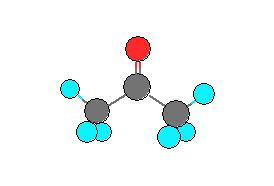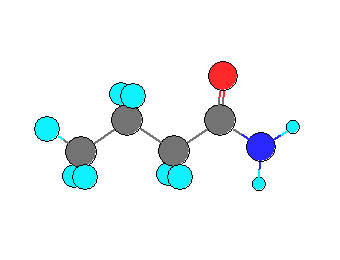# Second Challenge Problem 1

Problem 1 Summary

Part 1-1: Determine the value (and uncertainty) of the vapor pressure and heat of vaporization of acetone [CAS # 67-64-1] at these temperatures:
330, 375, 425, and 460 K

Acetone

Hill formula: C3H6O

Other names:
β-Ketopropane
2-Propanone
Allylic alcohol
Dimethyl ketone
Dimethylformaldehyde
Ketone propane
Ketone, dimethyl-
Methyl ketone
PropanonePart 1-2: Determine the value (and uncertainty) of the vapor pressure and heat of vaporization of butyramide [CAS # 541-35-5] at these temperatures:
415, 455, 490, and 520 K

Butanamide

Hill formula: C4H9NO

Other names:
Butanamide
Butanimidic acid
Butyramide
n-Butylamide
n-Butyramide
n-C3H7C(O)NH2Introduction

Vapor pressure is a fundamental thermodynamic property of a solid or liquid. It is the pressure generated at a particular temperature by pure component which has liquid (or solid) and vapor in equilibrium in a closed vessel. Its units are the usual units of pressure: engineering (psia, mm Hg (Torr), atm, bar, etc.) or SI units (Pa, kPa, MPa). The vapor pressure of a liquid increases with temperature between the triple point and the critical point.

Vapor pressure gives a measure of the escaping tendency of a material. The difference in vapor pressure between chemicals is the driving force for separation by distillation: The measure of separability for an ideal mixture of two components is the relative volatility, a(1 → 2), defined as,

a(1→2) = Psat1/Psat2 (1)

where Psat1 and Psat2 are the vapor pressures. Few liquid mixtures are ideal, so at low to moderate pressures this relative volatility equation usually contains activity coefficient terms, γi = fi/(xifi0), in which the vapor pressure is essentially the reference fugacity, fi0 ≈ Psati . The vapor pressure is also an important parameter in evaluating environmental and safety hazards of chemicals (e.g., evaporation rate, persistence in the soil, flash point, emissions, etc.) .

The most common vapor-pressure equation as a function of the temperature, T, is,

log(Psat) = A – B/(T+C) (2)

which was suggested by Antoine in 1888. High-accuracy use of the Antoine equation is restricted to a short range, typically 1 to 200 kPa. Thus the Antoine equation is often used for calculations related to atmospheric or vacuum distillations. The Antoine equation can cautiously be used to give vapor pressure data to ±15% over the temperature range where vapor pressure increases from 1 kPa to the critical pressure.

The Riedel equation is,

ln(Psat) = A + B/T + C ln(T) + D TN (3)

where A, B, C, D, and N are adjustable parameters (N is usually an integer from 1 to 6). The temperature, T, must be absolute temperature, K. The Riedel equation is used to fit data over the entire liquid range from the triple point to the critical point with a precision of 0.5 to 2%. It also has the correct derivative behavior (d(lnP)/d(1/T)) over most of the liquid range.

A second wide-range vapor-pressure equation is the Wagner equation which is given in reduced form: the temperature and pressure variables are divided by the critical temperature, Tc, and the critical pressure, Pc,

ln(Pr) = [a + b(1-Tr)1.5 + c(1-Tr)3 + d(1-Tr)6]/Tr  (4)

where Tr = T/Tc and Pr = Psat/Pc. There are many other vapor-pressure equations but the Antoine, Riedel, and Wagner equations are the three most commonly used in the chemical industry .

Heat of vaporization is the difference in heat content between coexisting liquid and vapor ;

ΔHvap = HV - HL  (5)

For a process where energy is absorbed or liberated at constant pressure, heat of vaporization is called enthalpy of vaporization. It usually has a maximum value at the triple point and decreases with temperature, going to zero at the critical point. Heat of vaporization is an extensive property and is usually reported in thermal units per mole (e.g., kJ/mol).

The heat of vaporization can be determined from direct calorimetric measurements (rare) or derived from accurate vapor pressure data via the Clapeyron equation:

ΔHvap = -RΔZVL [d(lnP)/d(1/T)]  (6)

where ΔZVL is the difference in compressibility factor (Z = PV/nRT) between coexisting vapor and liquid.

Assuming that the vapor phase behaves as an ideal gas (Z = 1) and the liquid phase compressibility factor is negligibly small, the Clapeyron equation reduces to the Clausius-Clapeyron equation:

ΔHvap = -R[d(LnP)/d(1/T)]  (7)

Heat of vaporization is an important quantity in the design of heat exchangers and other chemical process units. Enthalpy of vaporization is also used to calculate the Hildebrand solubility parameters that are useful in solvent selection to determine the mutual compatibility of chemicals .

Experimental techniques for vapor pressure and heat of vaporization measurements have been reviewed e.g. in [3-4]. Most commercial or in-house apparatus are typically suitable for routine measurements of vapor-liquid equilibria at temperatures between ca. –30° to 200° C and pressures between ca. 0.1 kPa to less than 10 MPa. Beyond these ranges, specialized apparatus are necessary and the cost of the measurement increases significantly. In recent years, there are a growing number of industrially important chemicals whose vapor pressures and heats of vaporization need to be determined outside of the range of the ordinary apparatus (e.g., highly volatile compounds at closely subcritical conditions, low volatile compounds with high melting points, etc.). Molecular simulation could offer an attractive alternative to these difficult laboratory measurements.

Problem Definition

Part 1-1: Determine the vapor pressure and heat of vaporization of acetone [67-64-1] at these temperatures:
330, 375, 425, and 460 K

Also specify the uncertainty in the reported values.

Part 1-2: Determine the vapor pressure and heat of vaporization of butyramide [541-35-5] at these temperatures:
415, 455, 490, and 520 K

Also specify the uncertainty in the reported values.

Problem Requirements and Restrictions:

1. Any force-field parameters (published or unpublished) may be used as long as they do not violate restriction 2 below and are reported in the manuscript submitted to the contest committee. Any force-field parameters published in a peer-reviewed journal by the date of the Contest announcement may be used, regardless of what experimental data were used to derive them.

2. Any publicly-available physical property data may be used to fit new force-field parameters, excluding vapor pressure and heat of vaporization data at temperatures above 303 K for acetone and for butyramide.

3. Computing vapor pressures at any set of temperatures, using a vapor pressure equation (such as those described in the Introduction) to fit the simulation data, and then calculating answers at the Contest state points is a permitted strategy.

4. Any method, direct or indirect, may be used to determine the heat of vaporization from the results of the simulation. If the Clapeyron equation is used as an indirect method (instead of the Clausius-Clapeyron equation), the densities of the coexisting saturated liquid and saturated vapor phases must be determined from the simulation.

Judging criteria:

The Problem 1 numerical score will be a maximum of 100 points based on the weighted numerical percentage difference for the vapor pressure and heat of vaporization as described below:

Vapor pressure

A numerical score will be computed as the sum of the absolute values of the percentage difference for each of four state points from the experimental benchmark data (the benchmark data state points will include upper and lower uncertainty bounds so that answers within the uncertainty bounds will be given a “0” difference). The total score will be the weighted average of Part 1-1 (weight = 30) and Part 1-2 (weight = 70) subtracted from 100. A perfect score is 100.

Heat of Vaporization

A numerical score will be computed as the sum of the absolute values of the percentage difference for each of four state points from the benchmark data determined by ASTM Standard Practice E 2071  (the benchmark data state points will include upper and lower uncertainty bounds  so that answers within the uncertainty bounds will be given a “0” difference). The total score will be the weighted average of Part 1-1 (weight = 30) and Part 1-2 (weight = 70) subtracted from 100. A perfect score is 100.

For a given state point, no credit is given if it is not calculated or deviates by more than 50 % from the corresponding experimental value. The final numerical score is the sum of the vapor pressure and the heat of vaporization scores.

In order to illustrate how the scoring will be done a Microsoft Excel spreadsheet (evaluation_sheet_1.xls) is provided. The spreadsheet contains pseudo data. The actual experimental data will be available in the spreadsheet after the close of the competition which will allow any entrant to enter their results and compute the exact numerical score used by the judges.

References:

 Poling B., Prausnitz J.M., O’Connell J.P.: The Properties of Gases and Liquids, 5th Ed., McGraw Hill, New York, 2000.

 Lyman W.J., Reehl W.F., Rosenblatt D.H.: Handbook of Chemical Property Estimation Methods, American Chemical Society, Washington, D.C., 1990.

 Ambose D.: “Vapour Pressures”, Chapter 7 in Specialist Periodical Report, Chemical Thermodynamics, Volume 1; The Chemical Society, London, 1973.

 Majer V., Svoboda V., Pick J.: Heats of Vaporization of Fluids, Elsevier, Amsterdam, 1989.

 ASTM Practice E-2071: “Standard Practice for Calculating Heat of Vaporization or Sublimation from Vapor Pressure Data,” in Annual Book of ASTM Standards, Vol. 14.02, ASTM, West Conshohocken, PA, 2002.

 Taylor B. N., Kuyatt C. E.: “Guidelines for Evaluating and Expressing the Uncertainty of NIST Measurement Results,” NIST TN 1297, National Institute of Standards and Technology, Washington, D.C., 1994.